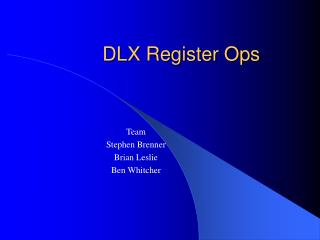DownloadDownload PresentationDLX Register Ops

# DLX Register Ops

Download Presentation## DLX Register Ops

- - - - - - - - - - - - - - - - - - - - - - - - - - - E N D - - - - - - - - - - - - - - - - - - - - - - - - - - -
##### Presentation Transcript

1. DLX Register Ops Team Stephen Brenner Brian Leslie Ben Whitcher

2. Instruction Types • I-type (immediate) • R-type (register to register) • J-type (jump)

3. Arithmetic & Logical Instructions • Arithmetic • Logical • Shift • Set-on-comparison

4. Arithmetic • ADD, SUB, MULT, DIV • Two registers. • ADDI, SUBI • Register & 16-bit immediate • ADDU, SUBU, MULTU, DIVU • Treats source register as unsigned integer. • ADDUI, SUBUI

5. Arithmetic examples • ADD R1, R2, R3 • Regs[R1] <- Regs[R2] + Regs[R3] • SUBI R1, R2, #2 • Regs[R1] <- Regs[R2] + 2

6. Logical • AND, OR, XOR, ANDI, ORI, XORI, LHI • LHI: Load High Immediate • Places 16-bit immediate into the most significant portion of destination register. • Fills remaining portion with 0s

7. Logical examples • AND R1, R2, R3 • Regs[R1] <- Regs[R2] & Regs[R3] • LHI R1, 0x42 • Regs[R1] <- 0x420000

8. Shift • SLL, SLLI • Shift Left Logical • Shifts contents of a register left by the number of bits specified by the other value • SRL, SRLI • Shift Right Logical • SRA, SRAI • Shift Right Arithmetic • Shifts contents of a register right. Keeps the same sign bit.

9. Shift examples • SLL R1, R2, R3 • Regs[R1] <- Regs[R2] << Regs[R3] • SRAI R1, R2, 0x2 • Regs[R1] <- Regs[R2] >> 0x2 • Keep R2’s sign

10. Set-On-Comparison • Sets the destination register to: • 1 when true, 0 when false

11. Set-on-Comparison examples • SLT R1, R2, R3 • If (Regs[R2] < Regs[R3]) Regs[R1] <- 1else Regs[R1] <- 0 • SNEI R1, R2, 0x7 • If (Regs[R2] != 0x7) Regs[R1] <- 1else Regs[R1] <- 0